## 26 Jan 2018

### Plumwood & Sylvan [Routley & Routley]. (6) ‘Negation and Contradiction,’ sect.6 “Negation as Otherthanness, and Progressive Modification of the Traditional Picture”, summary

[Search Blog Here. Index tabs are found at the bottom of the left column.]

[Central Entry Directory]

[Logic and Semantics, entry directory]

[Richard Sylvan (Francis Routley), entry directory]

[Val Plumwood (Val Routley), entry directory]

[Plumwood & Sylvan (Routley & Routley). Negation and Contradiction, entry directory]

[The following is summary. Boldface and bracketed comments are mine. This text is not proofread, so I apologize for its typos and other mistakes.]

Val Plumwood

(at that time as: Val Routley)

and

Richard Sylvan

(at that time as: Richard Routley)

6

Negation as Otherthanness, and Progressive Modification of the Traditional Picture

Brief summary:

(6.1) We will come to a notion of negation as restricted otherthaness, beginning in the logical philosophy of the 19th and early 20th centuries. Thus we will construct a Boole-Venn sort of semantics, where we assign values (taken very broadly as we will see) to atomic formulas, and the connectives further operate on those values. Quoting the authors:

Such an interpretation j is a mapping from (initial) wff of S to V which consists of a composite with (at least two) components, e.g. a geometrical area, a set, a mereological class, such that the following conditions are met:

j(~A) = V-j(A);

j(A & B) = j(A) ∩  j(b) i.e. the common part

j(A ∨ B) = j(A) ∪ j(B) i.e. the union (of areas)

A wff C of S is said to be BV-valid iff, for every mapping j, j(C) = V, i.e. the interpretation is always the whole of V.

(214)

There are three pertinent readings of the j interpretation function. (6.2) The first is the “geometrical reading.” It sees the j function as mapping to A some geometrical area or “territory”. (6.3) The second reading of j (the “set-theoretic reading”) sees it as mapping to A some set or class of objects, like “animal,” “plants,” and “horses.” The Boolean definitions of and and or are not problematic under this reading, but problems with not lead many who take this reading to use a non-Boolean interpretation of not. (6.4) The third reading of j is the “propositional reading.” It regards j as mapping to each wff some proposition or propositions. (6.5) The prevailing view of negation in the late 19th century is that it is restricted otherthanness, meaning that ~A is other than A, but not everything other than A. (6.6) To picture restricted otherthanness [under the geometrical reading], we need to think of ~A as being some other part of the terrain which is other than A’s part, but also not the entire remainder of that terrain. So it would be the situation in the right diagram and not the left: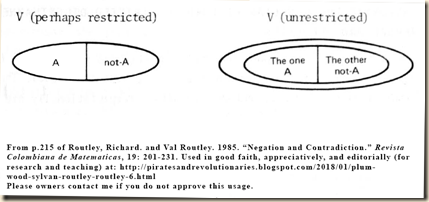We use the * operator on j to get this limited negation. Thus we have: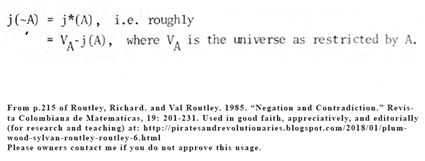(6.7) The picture we consider now is one where A and ~A are not mutually exclusive and also not exhaustive of the terrain.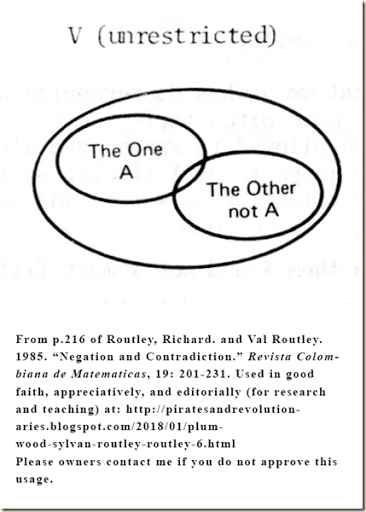Contents

6.1

[Boole-Venn Semantics]

6.2

[The Geometrical (“Territorial”) Reading of j]

6.3

6.4

6.5

[Late 19th Century Negation as Restricted Otherthanness]

6.6

[The * Operator on j for Restricted Negation]

6.7

[A and ~A as Non-Exclusive and Non-Exhaustive]

Summary

6.1

[Boole-Venn Semantics]

[To better examine otherthanness and negation in the 19th and early 20th centuries, we will examine Boole-Venn semantics, whose j interpretation function has three pertinent readings.]

[In section 5.3, we noted that the paraconsistent/relevant negation is the most “traditional” view on negation.] We will want to discuss the paraconsistent/relevant view of negation in terms of the notion of otherness and also within the historical context of nineteenth and early twentieth century logic. For this reason, we should construe certain structures using Boole and Venn. [The following ideas are technical, and I will probably misconstrue them, so please consult the quotation. My guess is that they mean the following. We will formulate these notions into a “Boole-Venn” interpretation, which would seem to involve sets. It seems that we will use A, B, and C to mean sentences. S seems to be the initial set of wffs, which would thus include A, B, C and so on. There is another set or mathematical entity called V. It seems like it could be a set of interpretation values or the space in a Venn diagram. We now will define the connectives. But to following the notation, let us first recall some concepts from Suppes’ Introduction to Logic. In section 1 and 2, he writes:

If A and B are sets, then by the intersection of A and B (in symbols: A B) we mean the set of all things which belong both to A and to B. Thus, for every x, x ∈ (AB) if and only if xA and xB; that is, symbolically:

(1)        (x)(x A B xA & xB).

If A is the set of all Americans, and B is the set of all blue-eyed people, then A B is the set of all blue-eyed Americans.
(Suppes, Introduction to Logic p.184)

If A and B are sets, then by the union of A and B (in symbols: AB) we mean the set of all things which belong to at least one of the sets A and B. Thus, for every x, x ∈ (A ∪ B) if and only if either x A or x B. (Notice that, as explained in Chapter 1, we use the connective ‘or’ in its non-exclusive sense: ‘xA or xB’ is false only in case both x A and x B.) Symbolically:

(2)        (x)(x ABx A xB).

If A is the set of all animals, and B is the set of all plants, then AB is the set of all living organisms. One often wishes to consider the union of two sets, however, even when they are not mutually exclusive. For instance, if A is the set of all human adults, and B is the set of all people less than 40 years old, then AB is the set of all human beings.
(Suppes, Introduction to Logic p.185)

In section 9.6, he writes (and note that Λ is the empty set):

we shall call D the domain of individ­uals, or sometimes the domain of discourse.

[...]

We shall denote the domain of individuals by ‘V’.

(Suppes, Introduction to Logic p.187)

When dealing with a fixed domain of individuals V, it is convenient to introduce a special symbol for the difference of V and a set A:

A = V∼A.

We call ∼A the complement of A. More generally, the difference BA of B and A is called the complement of A relative to B; so the complement of a set is simply its complement relative to the given domain of individuals.

As should be expected, we may find the complement of a set which itself results from operations on other sets. For example, let

V = {1, 2, 3}
A = {1, 2}
B = {2, 3}.

Then

A = V∼A = {1, 2, 3}∼{1, 2} = {3},

and correspondingly,

∼(A B) = V∼(A B) = {1, 2, 3}∼({1, 2} ∪ {2, 3})
= {1, 2, 3}∼{1, 2, 3} = Λ.
(Suppes, Introduction to Logic p.188)

And section 9.8:

one draws a rectangle to represent the domain of individuals, and then draws circles, or other figures, inside the rectangle – thinking of the points inside the various figures as corresponding to the members of the sets being represented by the figures.
(Suppes, Introduction to Logic p.195)

Now let us take a look at these Venn diagrams, as they will be helpful later. When two sets, A and B, are mutually exclusive sets [in section 9.5 he notates this as: A B = Λ] then we draw: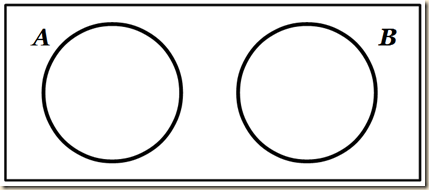(We might think of the cancellation model as being like this. When we conjoin A and ~A, each pushes the other out of the box, because the contents of the one exclude the other, and so long as A and B are conjoined as formulas, their spaces have been pushed out, or we might say, they force the other’s space to constrict down to nothing.) If A is a subset of B, A B, then: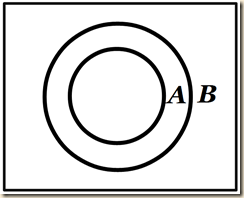(Here we note the same structure with Hospers: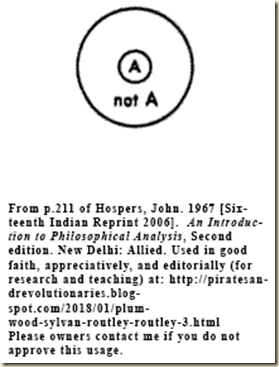So according to the explosion view, a formula and its negation completely exhaust the field of content. Their union is the full domain.) If we have A, B, and C and “A B (all A are B) and B C = Λ, (i.e., no B are C)” (196), then we draw: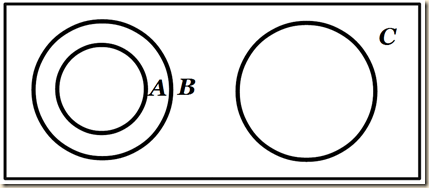We can also use shading to represent the empty set. This way, we can have an alternative way to make the first diagram above for A B = Λ: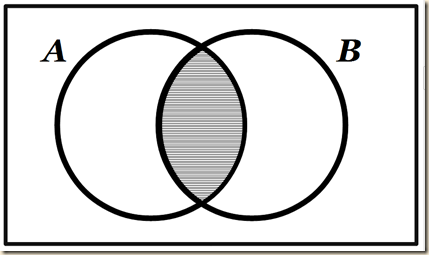This can instead mean that the intersection of A and B is the empty set, meaning that they share no members in common. And our second image above for A B can now instead be drawn: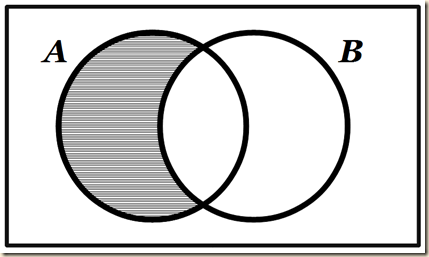It means that there is nothing in A that is outside of B. So now let us examine the rules for  evaluation for the connectives. We said that we have an initial set of wffs S. V is a composite set that can be a geometrical area, a set, and other things. And we have function j that maps initial wffs in S to whatever is in V. Let us begin by assuming that we just have A and ~A as our S formulas. We say that A and ~A completely divide up and exhaust all of V, like in the Hospers diagram:Here is the rule for evaluating negation:

j(~A) = V-j(A)

Let us take this apart, using a combination of Suppes and Hospers. Here is our diagram: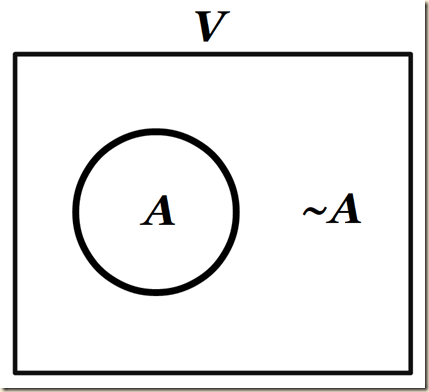j is a function that will map the formula letter to a space in the diagram. So j(A) is: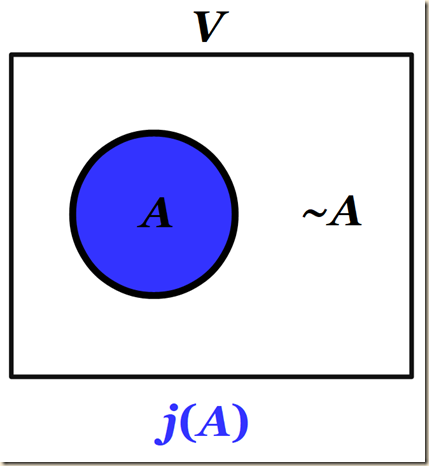Now, for V-j(A), perhaps we are to understand it simply as the subtraction of the spaces (I suppose it is the “difference of” V and A). So that would be: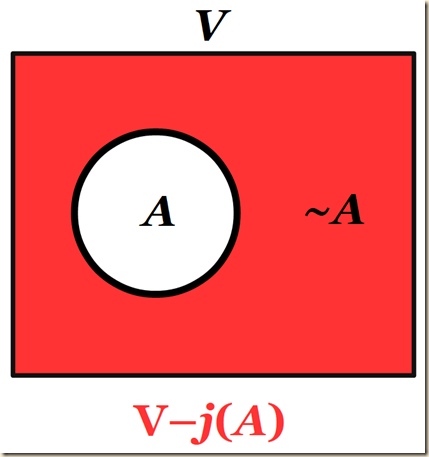But as we can see, that space is identical to j(~A):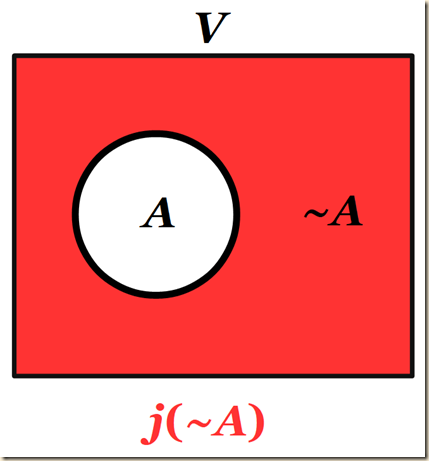So:

j(~A) = V-j(A)

This of course so far seems to be the classical situation. The conjunction is their union, which would here be the empty set, because they share nothing in common. No consider conjunction:

j(A & B) = j(A) ∩  j(b) i.e. the common part

Here I get a little confused. The intersection of A and ~A in the classical situation given above would be the empty set. But I thought the classical situation would yield full content, and instead be like union, which is here reserved for disjunction:

j(~A) = V-j(A);

j(A & B) = j(A) ∩  j(b) i.e. the common part

j(A ∨ B) = j(A) ∪ j(B) i.e. the union (of areas)

I am not sure how to get all this to work for the three theories, but as soon as I have something, I will try to lay it out. The next idea is about validity (it is called being BV-valid, which would seem to mean valid in this Boole-Venn system.) A formula is valid (and so I would think is a tautology) if for every j mapping, we get the whole of V. I would think then for example that A∨~A would be BV valid, because it will always yield the full V. But I am not sure. The next parts on soundness and completeness I do not quite get, but we discuss soundness and completeness of systems in Priest’s Introduction to Non-Classical Logic section 8.4.8. (To make a wild guess, perhaps the rules we use to make proofs can be obtained by using our semantics to find semantically valid formulas. But I am very wildly guessing as you can see.) The authors then write, “assignments under j may be reduced to assignments for initial wff only, and the conditions given used to definitionally extend the assignments to all wff.” I am guessing this means that j assigns the initial mappings to atomic formulas, and then the connectors are operations on those mappings (according to the rules above) rather than wholly new assignments. But I am not sure. The final point I think is that there are three particular interpretations of j that will interest us, and we address them in the following three paragraphs.]

In order to discuss the traditional idea that negation is otherthanness, and to consider negation in its historical setting, especially that of the nineteenth and earlier twentieth century work (when logic began its modern revival), it is helpful to introduce some of the ideas of Boole and Venn in an exact fashion. Consider, in particular a Boole-Venn interpretation of sentential logic S: such an interpretation can be extended to other logics, e. g. modal logics. Such an interpretation j is a mapping from (initial) wff of S to V which consists of a composite with (at least two) components, e.g. a geometrical area, a set, a mereological class, such that the following conditions are met:

j(~A) = V-j(A);

j(A & B) = j(A) ∩  j(b) i.e. the common part

j(A ∨ B) = j(A) ∪ j(B) i.e. the union (of areas)

A wff C of S is said to be BV-valid iff, for every mapping j, j(C) = V, i.e. the interpretation is always the whole of V. Then no doubt soundness and completeness may be established: a wff C is a theorem of S iff it is BV-valid. Further, assignments under j may be reduced to assignments for initial wff only, and the conditions given used to definitionally extend the assignments to all wff. What is of especial interest is however not the familiar results but the rendering, or intended interpretation, of function j. There are at least three overlapping groups of readings:

(214)

[contents]

6.2

[The Geometrical (“Territorial”) Reading of j]

[The first reading of j (the “geometrical reading”) sees it as mapping to A some geometrical area or “territory”.]

The first of the three readings of the j interpretation function is the that it maps A to a geometrical space, like in the Hospers diagram:This gives us a notion of the “territory” or area of A.

1. Geometrical reading with j(A), or |A| as it will sometimes be written, as what A covers (cf. Hospers above), or the area (or territory) of A.

(214)

[contents]

6.3

[The second reading of j (the “set-theoretic reading”) sees it as mapping to A some set or class of objects, like “animal,” “plants,” and “horses.” The Boolean definitions of and and or are not problematic under this reading, but problems with not lead many who take this reading to use a non-Boolean interpretation of not.]

The second is the set-theoretical reading of j. [Recall from section 3.8 our discussion of the Hospers passages and diagram. There we said that for Hospers, A can mean either some object or a predicate/proposition. Here it seems to mean something similar, but now it would seem to be some class of things, like horses, animals, plants. Routley and Routley say that under this reading, we have no problem with the Boolean definitions for conjunction and disjunction, but we encounter problems with negation. Perhaps we learn more later.]

2. Set-theoretical readings, with j(A) some set, e.g. the set of cases where A in true (i.e. the range of A). Set readings are central in the nineteenth century theories of complex terms – in the context of which negation was characteristically discussed. For this the wff of S are reconstrued as complex terns, -A as non-A (e.g. non-animal), A∨B as A or B (e.g. animal or plant) , etc. Then j(A) is the extension of A, e. g. j(horse) comprises (all) horses. While the operations of and and or are relatively straighforward in | forming complex terms, several logicians were distinctly worried about not and in fact opted, as we shall see, for a non-Boolean interpretation of not.

(214-215. Note, it is written “straighforward” in the original.)

[contents]

6.4

[The third reading of j (the “propositional reading”) regards it as mapping to each wff some proposition or propositions.]

The third type of reading of j are propositional readings. Here j(A) means some certain proposition or sum of propositions. [The authors next write “In particular, an obvious j function, exploited below, is that which maps each initial wff to the proposition it expresses. I do not know what the “obvious” means here, but perhaps we learn later. Otherwise the point seems to mean that to A we would map something like “snow is white” or whatever.]

3. Propositional readings, where j(A) is some proposition or sum of propositions. In particular, an obvious j function, exploited below, is that which maps each initial wff to the proposition it expresses.

(215)

[contents]

6.5

[Late 19th Century Negation as Restricted Otherthanness]

[The prevailing view of negation in the late 19th century is that it is restricted otherthanness, meaning that ~A is other than A, but not everything other than A.]

Routley and Routley note that in the late 19th century, the prevailing view was that negation applies to both terms [so I would suspect, to things like “animals,” “plants,” but I am not sure] and also to judgments. And, they saw negation as otherthanness [I am not sure I fully grasp this concept. It seems that negation simply means what is other to whatever is being negated.] [Now, the classical notion of negation was also otherthanness, and there was no restriction to that otherthanness. So ~A in this sense of unrestricted otherthanness is the entire remainder of the field outside A.] But the prevailing view of negation as otherthanness was that it was a restricted otherthanness [which might mean that ~A is other than A, but it is not everything other than A.] We see evidence of this restricted otherthanness of negation in Baldwin’s Encyclopedia, and we even see tendencies of it in Venn.

The late nineteenth century view was that negation, which applied to terms and also to judgements, is otherthanness, and on the prevailing view restricted otherthanness. Thus according to Baldwin’s Encyclopedia (p. 147) ‘Not-A = other than A – a second thing to A’. But it was not anything other than A. Joseph, for one, considers the view that ‘whatever it (the positive term) be, the negative term covers everything else’, and rejects it. His conclusion is that

A positive term and its corresponding negative (e.g. blue and not-blue) may then be said to divide between them not indeed the whole universe, but the limited universe of things, to which they belong (p. 44fn).15

Venn himself acknowledges such limits:

It is quite true that we always do recognize a limit, sometimes expressed but more often tacit, as to the extent over which not-X is to be allowed to range; and also we not infrequently do so in respect of X itself; so long as these expressions are set before us in words, and not in symbols only.

Though he continues, ‘Between them X and not-X must fill up the whole field of our logical enquiry’, he admits restricted fields, and allows that we can choose the universe (of discourse) – admissions that run him into serious trouble where different negative terms call for different restricted fields. Venn’s procedure and the Boolean picture captured in the j-functions then break down.

(215)

(15) Joseph elaborates his view through examples such as ‘intemperate’, ‘uneven’ and ‘not-blue’, e.g. the latter is equated with ‘coloured in some way other than blue’ (italics added). More generally, not-A will signify what a subject, which might be A, will be if it is not A’ (p.43).
(228)

[contents]

6.6

[The * Operator on j for Restricted Negation]

[To picture restricted otherthanness, we need to think of ~A as being some other part of the domain which is other than A’s part, but also not the entire remainder of that domain. We use the * operator on j to get this limited negation.]

So this notion of restricted otherthanness is not the explosive negation where whatever is the negation of A is everything else in the field. Rather, it is a restricted part of that field, as we see in the diagram:[I am a little bit confused by the notion of restrictedness. It seems that V is unrestricted when divisions in all its contents are not entirely dictated by any one negation. In other words, it is restricted when one negation can dictate the way the entire contents can be divided. I get confused because I thought we were calling the otherness in the right diagram a restricted otherthanness. Were that so, then V is unrestricted but ~A is restricted.] To obtain a more precise formulation for negation in terms of the j function that would render a limited otherthaness, we need an additional operation [or maybe function] *.[I would think that *j would be whatever the restricted otherthaness is. So in the right diagram above, j*(A) would take “The one A” or just A as the input, and the output would be “The other not-A”, which is like the other colors other than blue, when A is blue, but not every other thing whatsoever. Yet with the symbolic formulation, I am not sure I get that meaning from it. In particular, I do not know what “the universe as restricted by A” means. It cannot mean, “everything that is not A”. For, that would just give us the left diagram, which we do not want. In terms of the spaces shown in the right diagram, VA would seem to be the space surrounding both the A and its limited other. If that is not the meaning of “the universe as restricted by A”, then I am confused.] Routley and Routley then note some of the properties of the * operator/function. It is involutary [wiki], meaning that when applied a second time you get the original. And from a certain view (British perceptions [I do not know what they are]), the union of j with *j is the empty set [meaning that they share nothing in common,] and thus j and *j are exclusive.

It is by now more or less clear how to repair matters. The picture on the left gives way to the picture on the right:In functional terms it is a little more complex, but again now evident enough. A further operation * is added to the modelling and the rule for negation amended to:|

Some of the intended properties of * are evident enough, e.g. j** = j, i.e. * is involutary, and on British perceptions j ∩ j* = Λ, i.e. j and j* are always exclusive.

(215-216)

[contents]

6.7

[A and ~A as Non-Exclusive and Non-Exhaustive]

[The picture we consider is one where A and ~A are not mutually exclusive and not exhaustive of the domain.]

Hegel shows that there are important cases where negation is not exclusive. For, in Hegel’s philosophy, we have special cases where A = not-A, and for that to hold, A cannot exclude its negation. But we do not need to take such a radical position as this. Our concern here will be primarily with situations where A and ~A hold, on account of their not being mutually exclusive. So we find for example in Simone de Beauvoir the notion that “presence and absence are not mutually exclusive, or that what A covers does not fully exclude what ~A covers” (qtg R&R but paraphrasing de Beauvoir, I assume). We now instead have a terrain picture with the following structure:[The idea here seems to be that not only do A and ~A not exhaust the entire terrain, also, they can overlap somehow. This would seem to be unlike the * operator above which says the intersection of A and ~A would be an empty set.] Next we will picture A and ~A as lying back to back, like a phonograph.

To the resulting picture Hegel added, in effect, a significant liberalizing element – one that is forced if contraposition principles (etc.) are to be duly respected, especially at the statement stage – namely that exclusiveness is not always guaranteed. The reason is, according to Hegel, that for certain A (of categorial type) A = not-A. It is not however necessary to accept, for anything that follows, this difficult doctrine: it would be nearer the mark to say that what is supposed is that there are situations where A↔~A, and better to say, still less demandingly, where both A and ~A hold. It is enough to say, with Simone de Beauvoir (p.18) that presence and absence are not mutually exclusive, or that what A covers does not fully exclude what ~A covers. The liberalized picture which emerges is important:Given that j* need not exclude j, the rule j(~A) = j*(A) again suffices (equivalently, and revealing more structure, j(~A) – V-j̅(A), where j̅ is the inverse of j: see RLR, 13.5). To glance ahead, we shall simply put The One and The Other back to back, as in a phonograph record, and we will have, more or less, the sought picture of negation.

(216)

[contents]

From:

Routley, Richard. and Val Routley. 1985. “Negation and Contradiction.” Revista Colombiana de Matematicas, 19: 201-231.

Sources cited by the authors:

 S. de Beauvoir, The Second Sex, Four Square, London, 1960.

 H.W.B. Joseph, An Introduction to Logic, Clarendon, London, 1916.

“RLR”, the abbreviation for: R. Routley, R.K. Meyer, V. Plumwood and R.T. Brady, Relevant Logics and Their Rivals, Ridgeview Publishing Company, Atascadero, California, 1983.

Suppes, Patrick. 1957. Introduction to Logic. New York: Van Nostrand Reinhold / Litton Educational.

URL for this entry:

http://piratesandrevolutionaries.blogspot.com/2018/01/plumwood-sylvan-routley-routley-6.html

.